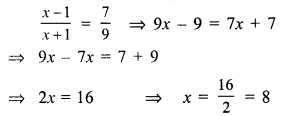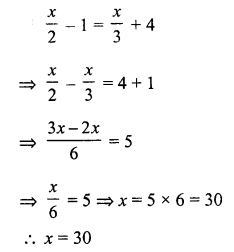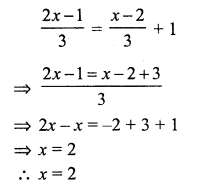# RS Aggarwal Class 7 Solutions Chapter 7 Linear Equations in One Variable Ex 7C

In this chapter, we provide RS Aggarwal Solutions for Class 7 Chapter 7 Linear Equations in One Variable Ex 7C for English medium students, Which will very helpful for every student in their exams. Students can download the latest RS Aggarwal Solutions for Class 7 Chapter 7 Linear Equations in One Variable Ex 7C Maths pdf, free RS Aggarwal Solutions Class 7 Chapter 7 Linear Equations in One Variable Ex 7C Maths book pdf download. Now you will get step by step solution to each question.

### RS Aggarwal Solutions for Class 7 Chapter 7 Linear Equations in One Variable Ex 7C Download PDF

Question 1.
Solution:
(d)Question 2.
Solution:
(d)Question 3.
Solution:
(a)
2n + 5 = 3 (3n – 10)
⇒ 2n + 5 = 9n – 30
⇒ 9n – 2n = 5 + 30
⇒ 7n = 35
⇒ n = 5

Question 4.
Solution:
(c)Question 5.
Solution:
(c)
8 (2x – 5) – 6 (3x – 7) = 1
⇒ 16x – 40 – 18x + 42 = 1
⇒ -2x + 2 = 1
⇒ -2x = 1 – 2 = -1
x = 12

Question 6.
Solution:
(d)Question 7.
Solution:
(a)Question 8.
Solution:
(b)
Let first whole number=x
Then second number = x + 1
and sum = 53
x + x + 1 = 53
⇒ 2x = 53 – 1
⇒ 2x = 52
⇒ x = 26
Smaller number = 26

Question 9.
Solution:
Let first even number = 2x
Then second number = 2x + 2
and sum = 86
2x + 2x + 2 – 86
⇒ 4x = 86 – 2 = 84
⇒ x = 21
Larger even number = 2x + 2 = 2 x 21 + 2 = 42 + 2 = 44

Question 10.
Solution:
(b)
Let first odd number = 2x + 1
Second number = 2x + 3
2x + 1 + 2x + 3 = 36
⇒ 4x + 4 = 36
⇒ 4x = 36 – 4 = 32
⇒ x = 8
Smaller number = 2x + 1 = 2 x 8 + 1 = 16 + 1 = 17

Question 11.
Solution:
(d)
Let number = x
2x + 9 = 31
⇒ 2x = 31 – 9 = 22
⇒ x = 11

Question 12.
Solution:
(a)
Let number = x then
3x + 6 = 24
⇒ 3x = 24 – 6 = 18
⇒ x = 6
Number = 6

Question 13.
Solution:
(a)Question 14.
Solution:
(b)
Let first angle = x
Then second = 90° – x
x – (90° – x) = 10
⇒ x – 90° + x = 10°
⇒ 2x = 10° + 90° = 100°
x = 50°
Second angle = 90° – 50° = 40°
Larger angle = 50°

Question 15.
Solution:
(b)
Let first angle = x
Then second = 180° – x
x – (180° – x) = 20°
⇒ x – 180° + x = 20°
⇒ 2x = 20° + 180° = 200°
x = 100°
Second angle = 180° – 100° = 80°
Smaller angle = 80°

Question 16.
Solution:
(c)
Let age of A = 5x
Then age of B = 3x
After 6 years,
A’s age = 5x + 6
and B’s age = 3x + 6
5x+63x+6 = 75
⇒ 25x + 30 = 21x + 42
⇒ 25x – 21x = 42 – 30
⇒ 4x = 12
⇒ x = 3
A’s age = 5x = 5 x 3 = 15 years

Question 17.
Solution:
(b)
Let the number = x
According to the condition,
5x = 80 + x
⇒ 5x – x = 80
⇒ 4x = 80
⇒ x = 20
Number = 20

Question 18.
Solution:
(c)
Let width of rectangle = x m
Then length = 3x m
Perimeter = 96 m
2 (x + 3x) = 96
⇒ x + 3x = 962 = 48
⇒ 4x = 48
⇒ x = 12
Length = 3x = 12 x 3 = 36 m

All Chapter RS Aggarwal Solutions For Class 7 Maths

—————————————————————————–

All Subject NCERT Exemplar Problems Solutions For Class 7

All Subject NCERT Solutions For Class 7

*************************************************

I think you got complete solutions for this chapter. If You have any queries regarding this chapter, please comment on the below section our subject teacher will answer you. We tried our best to give complete solutions so you got good marks in your exam.

If these solutions have helped you, you can also share rsaggarwalsolutions.in to your friends.